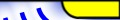Index    Chapter 1    Chapter 2    Chapter 3    Conclusion    Appendix    Matlab Code    Audio DemoChapter 2.

An OFDM system was modelled using Matlab to allow various parameters of the system to be varied and tested. The aim of doing these simulations was to measure the performance of OFDM under different channel conditions, and to allow for different OFDM configurations to be tested. Four main criteria were used to assess the performance of the OFDM system, which were its tolerance to multipath delay spread, peak power clipping, channel noise and time synchronization errors.

## 2.1 OFDM Model Used

The OFDM system was modelled using Matlab and is shown in Figure 16. A brief description of the model is provided below.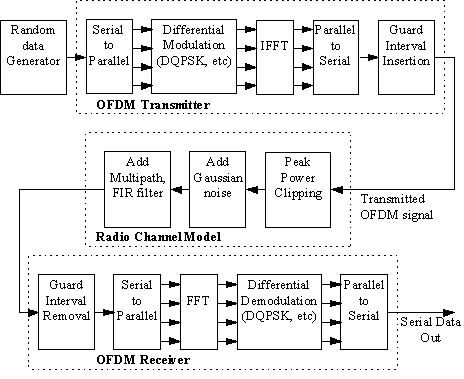Figure 16 OFDM Model used for simulations

### 2.1.1 Serial to Parallel Conversion

The input serial data stream is formatted into the word size required for transmission, e.g. 2 bits/word for QPSK, and shifted into a parallel format. The data is then transmitted in parallel by assigning each data word to one carrier in the transmission.

### 2.1.2 Modulation of Data

The data to be transmitted on each carrier is then differential encoded with previous symbols, then mapped into a Phase Shift Keying (PSK) format. Since differential encoding requires an initial phase reference an extra symbol is added at the start for this purpose. The data on each symbol is then mapped to a phase angle based on the modulation method. For example, for QPSK the phase angles used are 0, 90, 180, and 270 degrees. The use of phase shift keying produces a constant amplitude signal and was chosen for its simplicity and to reduce problems with amplitude fluctuations due to fading.

### 2.1.3 Inverse Fourier Transform

After the required spectrum is worked out, an inverse fourier transform is used to find the corresponding time waveform. The guard period is then added to the start of each symbol.

### 2.1.4 Guard Period

The guard period used was made up of two sections. Half of the guard period time is a zero amplitude transmission. The other half of the guard period is a cyclic extension of the symbol to be transmitted. (As discussed in section 1.3.10). This was to allow for symbol timing to be easily recovered by envelope detection. However it was found that it was not required in any of the simulations as the timing could be accurately determined position of the samples.

After the guard has been added, the symbols are then converted back to a serial time waveform. This is then the base band signal for the OFDM transmission.

### 2.1.5 Channel

A channel model is then applied to the transmitted signal. The model allows for the signal to noise ratio, multipath, and peak power clipping to be controlled. The signal to noise ratio is set by adding a known amount of white noise to the transmitted signal. Multipath delay spread then added by simulating the delay spread using an FIR filter. The length of the FIR filter represents the maximum delay spread, while the coefficient amplitude represents the reflected signal magnitude.

The receiver basically does the reverse operation to the transmitter. The guard period is removed. The FFT of each symbol is then taken to find the original transmitted spectrum. The phase angle of each transmission carrier is then evaluated and converted back to the data word by demodulating the received phase. The data words are then combined back to the same word size as the original data.

### 2.1.7 OFDM simulation parameters

Table 9 shows the configuration used for most of the simulations performed on the OFDM signal. An 800-carrier system was used, as it would allow for up to 100 users if each were allocated 8 carriers. The aim was that each user has multiple carriers so that if several carriers are lost due to frequency selective fading that the remaining carriers will allow the lost data to be recovered using forward error correction. For this reason any less then 8 carriers per user would make this method unusable. Thus 400 carriers or less was considered too small. However more carriers were not used due to the sensitivity of OFDM to frequency stability errors. The greater the number of carriers a system uses, the greater it required frequency stability.

For most of the simulations the signals generated were not scaled to any particular sample rate, thus can be considered to be frequency normalized. Three carrier modulation methods were tested to compare their performances. This was to show a trade off between system capacity and system robustness. DBPSK gives 1 bits/Hz spectral efficiency and is the most durable method, however system capacity can be increased using DQPSK (2 bits/Hz) and D16PSK (4 bits/Hz) but at the cost of a higher BER. The modulation method used is shown as BPSK, QPSK, and 16PSK on all of the simulation plots, because the differential encoding was considered to be an integral part of any OFDM transmission.

(Addendum 10/2001: Many OFDM systems now use coherent modulation instead of differential modulation as coherent modulation allows the use of Quadrature Amplitude Modulation (QAM) carrier modulation, which improves the spectral efficiency).

 Parameter Value Carrier Modulation used DBPSK, DQPSK, D16PSK FFT size 2048 Number of carrier used 800 Guard Time 512 samples (25%) Guard Period Type Half zero signal, half a cyclic extension of the symbol

Table 9 OFDM system parameters used for the simulations

(Errata Note: 10/2001. The simulation results presented here show the overall Symbol Error Rate (SER) rather than the Bit Error Rate (BER) as indicated on the graph results and discussions. For BPSK the SER equals the BER, however for QPSK the BER will be approximately half the SER. This is because two bits of information are transferred for each QPSK symbol and typically only single bit errors occur when suitable mapping is used (gray coding) and the noise level is low. This approximation is valid for a SER below approximately 1x10-2. For 16PSK the same argument applies, thus the true BER is approximately one quarter the SER).

## 2.2 OFDM Simulated Results

### 2.2.1 Multipath Delay Spread Immunity

For this simulation the OFDM signal was tested with a multipath signal containing a single reflected echo. The reflected signal was made 3 dB weaker then the direct signal as weaker reflections then this did not cause measurable errors, especially for BPSK. Figure 17 shows the simulation results.

It can be seen from Figure 17 that the BER is very low for a delay spread of less then approximately 256 samples. In a practical system (i.e. one with a 1.25 MHz bandwidth) this delay spread would correspond to ~80 m sec. This delay spread would be for a reflection with 24 km extra path length. It is very unlikely that any reflection, which has travelled an extra 24 km, would only be attenuated by 3 dB as used in the simulation, thus these results show extreme multipath conditions. The guard period used for the simulations consisted of 256 samples of zero amplitude, and 256 samples of a cyclic extension of the symbol. The results show that the tolerable delay spread matches the time of the cyclic extension of the guard period. It was verified that the tolerance is due to the cyclic extension not the zeroed period with other simulations. These test however are not shown to conserve space.Figure 17 Delay Spread tolerance of ODFM

For a delay spread that is longer than the effective guard period, the BER rises rapidly due to the inter-symbol interference. The maximum BER that will occur is when the delay spread is very long (greater then the symbol time) as this will result in strong inter-symbol interference.

In a practical system the length of the guard period can be chosen depending on the required multipath delay spread immunity required.

### 2.2.2 Peak Power Clipping

It was found that the transmitted OFDM signal could be heavily clipped with little effect on the received BER. In fact, the signal could the clipped by up to 9 dB without a significant increase in the BER. This means that the signal is highly resistant to clipping distortions caused by the power amplifier used in transmitting the signal. It also means that the signal can be purposely clipped by up to 6 dB so that the peak to RMS ratio can be reduced allowing an increased transmitted powerFigure 18 Effect of peak power clipping for OFDM

### 2.2.3 Gaussian Noise Tolerance of OFDM

It was found that the SNR performance of OFDM is similar to a standard single carrier digital transmission. This is to be expected, as the transmitted signal is similar to a standard Frequency Division Multiplexing (FDM) system. Figure 1 shows the results from the simulations. The results show that using QPSK the transmission can tolerate a SNR of >10-12 dB. The bit error rate BER gets rapidly worse as the SNR drops below 6 dB. However, using BPSK allows the BER to be improved in a noisy channel, at the expense of transmission data capacity. Using BPSK the OFDM transmission can tolerate a SNR of >6-8 dB. In a low noise link, using 16PSK can increase the capacity. If the SNR is >25 dB 16PSK can be used, doubling the data capacity compared with QPSK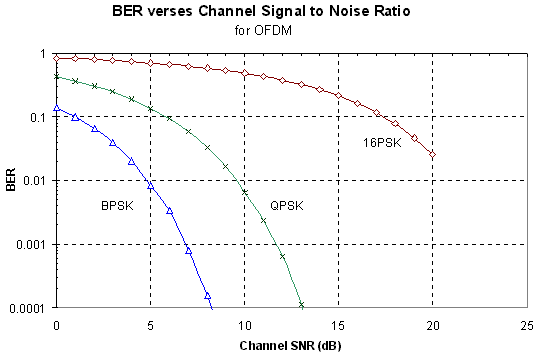Figure 19 BER verse SNR for OFDM using BSPK, QPSK and 16PSK

(Errata 10/2001: The simulation results shown in Figure 19 are slightly incorrect due to the calculation of the noise level in the simulation. The simulations shown above calculated the signal to noise ratio based on the power of the time domain signal waveform and the power of the time domain noise waveform, with no consideration of the signal bandwidth. At the receiver the signal is filtered by the FFT stage, thus making the receiver only see noise within the signal bandwidth. The simulations were performed using 800 carriers and generated using a 2048-point IFFT. The nyquist bandwidth is half the transmission sample rate as the signal is real (i.e. no imaginary components) and so the nyquist bandwidth corresponds to 1024 carriers. The signal bandwidth is thus 800/1024 = 0.781 or 78.1% of the nyquist bandwidth. Since the receiver was only seeing 78.1% of the total noise the error rate is lower than it should be. The correct SNR values can be found by adding 1.07 dB (10.log10(0.781)) to the scale in Figure 19. Also note the simulation results actually show the Symbol Error Rate rather than the Bit Error Rate).

### 2.2.4 Timing Requirements

One of the big questions at the start of the thesis was how tolerant OFDM would be to a starting time error. The problem was that when an OFDM receiver is initially switched on it will not be synchronized with the transmitted signal. So a synchronization method was required. The proposed method was that the OFDM signal could be broken up into frames, where each frame transmits a number of symbols (somewhere between 10-1000). At the start of each frame a null symbol is transmitted, thus allowing the start of the frame to be detected using envelope detection. However using envelope detection only allows the start to be detected to within a couple of sample, depending on the noise in the system. It was not known whether this timing accuracy was sufficient. This method was used for the synchronization in the practical tests performed. (See section 2.3)

Figure 20 shows the effect of start time error on the received BER. This shows that the starting time can have an error of up to 256 samples before there is any effect of the BER. This length matches the cyclic extension period of the guard interval, and is due to the guard period maintaining the orthogonality of the signal

In any practical system, the timing error made be either early or late, thus any receiver would aim for the middle of the expected starting time to allow for an error of ±128 samples. In addition, if the signal is subject to any multipath delay spread, this will reduce the effective stable time of the guard period, thus reducing the starting time error tolerance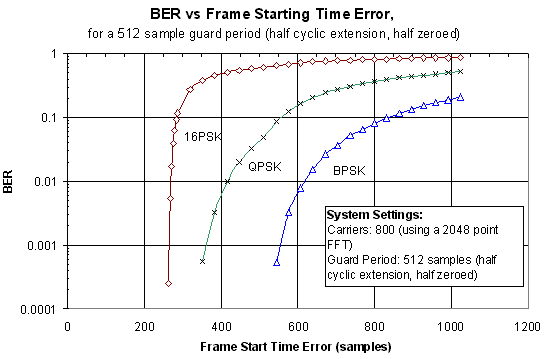Figure 20 Effect of frame synchronization error on the received OFDM signal.

## 2.3 Practical Measurements

A set of practical measurements was made on the OFDM system. This was done so that the simulated results could be partly verified and so that difficulties in implementing a practical system could be tackled, and to measure some effects which were hard to simulate.

The practical measurements were performed using a Personal Computer (PC) as an OFDM generator and receiver. A Matlab program was developed to process the input and output signals and a Sound Blaster 16 card was used to play the transmitted OFDM signal, and used to record the received signal. Only one PC was available with a sound card and so the transmission was performed in two steps. The transmitted signal was generated using Matlab then played out the sound card and recorded onto an audio recorder. This signal was then played back and re-recorded by the sound card on the computer. The received signal was then processed using a Matlab script. Two different audio recorders were tested, as each gave a different performance.

The first used was the HiFi stereo audio track on a high quality VCR (Panasonic Super-VHS FS90). This gave a high quality audio channel (SNR >90dB, 20Hz - 20kHz range, and crystal accuracy stability of frequency (0.005%)), which was a good model for a near perfect radio channel

The system was also tested using an audio tape player. The tape player had a much poorer performance, in noise (~50dB SNR), audio bandwidth (20Hz-15kHz), and particularly frequency stability (2%). This provided a good test of the performance of OFDM in a channel with very poor frequency stability

### 2.3.1 Extended Model

The basic OFDM model used for the simulations (see section 2.1) was extended to allow for the received signal to be automatically be synchronized to the OFDM frame structure, and for large data files to be able to be transmitted.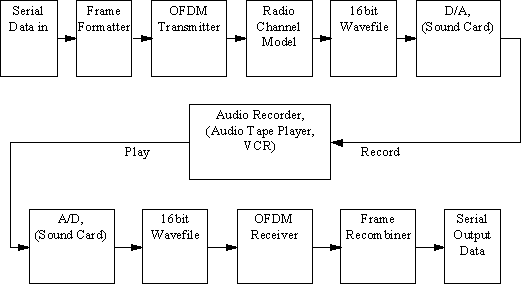### 2.3.2 Transmission Protocol

A basic frame structure was used to allow the receiver to synchronize with the transmitted signal. The frames were marked out by having a null symbol (zero amplitude) between frames (see Figure 21), allowing the start of each frame to be detected using an envelope detector in software. The transmitted signal consisted of a number of frames (typically 1- 100), with a preamble at the start and a post signal at the end (see Figure 22). The preamble was used to provide the start time of the first frame and consisted on a mixture of tones. This was required so that the envelope detector had a signal to initialise the filtering required.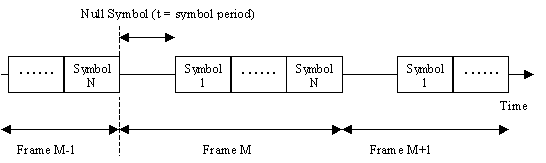Figure 21 Frame Structure, showing the null symbol between frames

The envelope detector was implemented by rectifying the input signal then applying a moving average filter to the signal. The length of the filter was made exactly the same length as the null symbol. This results in the filtered signal having a minimum amplitude at the start of a frame. This minimum was used to find the starting location in which to decode the entire frame. Each frame consists of a number of symbols (typically 5-40) that contain the actual data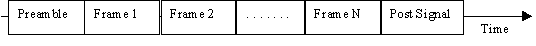Figure 22 Frame Structure used for the OFDM transmission

### 2.3.3 Video Recorder

The first simulated channel used was the audio track of a VCR

#### 2.3.3.1 Number of Carriers Used

For an OFDM system, the number of carriers used in the transmission determines several parameters about the system. These include the processing speed required, the symbol time (thus the maximum delay spread that can be tolerated), the number of users the available bandwidth can be split over (i.e. one carrier per user), and the frequency stability required.

Since there was no easy way of simulating this effect with the Matlab model developed, it was decided to measure it in a practical way. This was done by varying the number of carriers used when transmitting the OFDM over the link simulated by the VCR. The frequency stability of the VCR’s recording and playing was considered to be approximately the same for each of the tests performed. This allowed the relative effect of varying the number of carriers for a fixed frequency stability.

The data used in the transmission was that of a grey-scale bit-map image of a fish. The original of the image is show below in Figure 23Figure 23 Image used in transmission tests

The transmission was sent using 256PSK. This was chosen because it gave the highest transmission efficiency (~8 bits/Hz), thus resulting in the smallest transmission data size. By sending each 8-bit grey scale pixels as one carrier per symbols, any phase errors in the transmission directly correspond to a change in the intensity of the received signal. This allows the phase angle errors to be judged from the image quality. Since the phase error due to a frequency offset or phase noise has the same effect on the received data as gaussian noise, it can be effectively converted to an equivalent signal to noise ratio of the received image.

The image quality and phase error was measured at different number of carriers used to transmit the signal.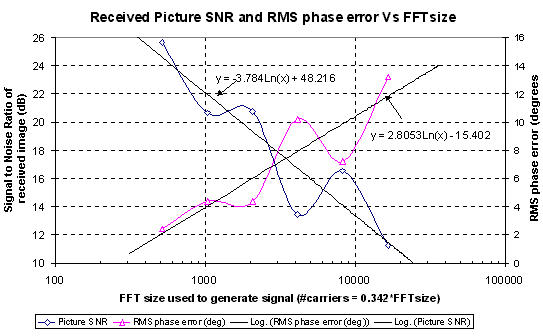Figure 24 Performance of the OFDM using the VCR as a channel, as a function of the number of carriers used

Figure 24 show the effect that increasing the number of carriers had on the received signal. It can be seen that the larger the FFT size (and number of carriers used), the worse the performance of the system. This is due to the VCR having a fixed frequency stability thus the closer the carriers are in the transmission, the worse the effect of the frequency error. The SNR of the received image for an FFT size of 2048 was only 20 dB. This is much worse then the SNR ratio that would be expected from the channel noise. The VCR has excellent noise performance (>70-90 dB SNR for gaussian noise) however this limit is no where near reached due to frequency stability problems causing an effective SNR of between 10-3 0dB. This indicates that the performance of the system is not limited by the gaussian noise of the system, but the frequency stability

Since the frequency stability of the is such a problem in an practical radio OFDM system the receiver would have to be frequency locked to the transmitter in order achieve the maximum performance

 Received Image from VCR audio channel Error in received image (contrast enhanced to show the errors)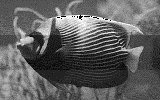16384 point FFT, 5600 carriers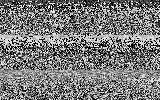16384 point FFT, 5600 carriers256 point FFT, 75 carriers256 point FFT, 75 carriers

Table 10 Received OFDM images using the audio channel of a VCR

Table 10 shows some of the received images that were used to generate Figure 24. It can be seen that the image transmitted using 5600 carriers has bands in the image, due to the phase errors (and thus pixel intensity errors) in the transmission. Also some of the pixels that are white (on the top of the fish) have wrapped round to show white. This is due to the received phase error causing a wrap around from 255 to 0 in intensity

### 2.3.4 Peak OFDM Performance for the VCR link

After trying out different OFDM system parameters such as the number of carriers used, system bandwidth and guard period length, it was found that very high spectral efficiency could be achieved. Figure 25 shows the maximum performance that could be achieved on the VCR audio track.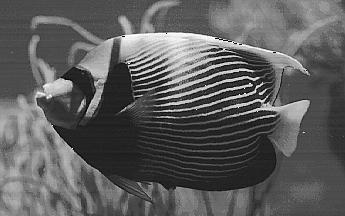Figure 25 Image transferred at 134kbps in an 18.2kHz bandwidth on the VCR audio channel, using 210 carriers.

The image of the fish was transferred using 256PSK. The total transmission time was 4.54 seconds for 76246 bytes of data, with only 18.2 kHz bandwidth. This gives a spectral efficiency of 7.4 bits/Hz. This is just under the theoretical limit of 8 bits/Hz for 256PSK and is due to overhead in the guard period and frame symbols. The signal was generated using a 512-point FFT, using 210 carriers, and a guard period of 32 samples. The carriers used were based on the frequency response of the VCR link measured in section 2.3.4.2, so that the maximum bandwidth could be used

The received image in Figure 25 has slight phase errors, which are just noticeable as bands in the image. Also some pixel on the top fin of the fish have wrapped around from white to black.

#### 2.3.4.1 Peak Power Clipping

The clipping tolerance of the OFDM signal was tested to verify that OFDM can handle a large amount of peak power clipping before any significant increase in the bit error rate (BER) occurs. The simulations indicated that OFDM could handle up to 9 dB of clipping (for QPSK) before the BER became detectable. This result was slightly surprising as any non-linearity in the system lead to intermodulation distortion. Thus the initial expectation was that OFDM would be susceptible to any clipping of the signal

The clipping of the signal was performed during the recording from the VCR back to the computer for decoding. The clipping of the signal was achieved by using back- to-back germanium diodes across at the output of the VCR with a resistor in series with the VCR to limit the current flow. The signal was observed on a CRO and increased in amplitude until clipping occurred. The peak power clipping was measured by finding the ratio of the peak signal level before clipping, to the peak signal level after clipping

 Peak Signal before clipping  (Vp-p) Peak Signal after clipping (Vp-p) Calculated Peak Power Clipping (dB) Measured BER of received signal Predicted BER from simulation 1.45 0.72 6.08 <0.00006 1.88 0.80 7.45 <0.00006 2.01 0.805 7.95 <0.00006 2.65 0.853 9.85 0.0004 <0.00009 3.55 0.917 11.8 0.0036 0.0038 4.6 0.935 13.84 0.0125 0.0208

Table 11 Results of clipping the OFDM signal, showing the resulting BER

Table 11 shows the measured error rates when the signal was clipped, and the expected BER based on the simulations. The BER was found to be too small (<0.00006) for peak power clipping up to 8 dB. The BER was only detectable for peak power clipping of >8-10 dB, matching the expected result of 9 dB measured from the simulations (see section 2.2.2). For high levels of clipping, from 12- 14 dB, the measured BER was actually lower then the simulated results. This is probably due to the fact that the germanian diodes used for clipping of the signal were not clipping as abruptly as in the simulation resulting in lower intermodulation distortion and a lower BER.

#### 2.3.4.2 VCR performance

The audio performance of the VCR link was measured so that the quality of the channel used for the practical measurements could be assessed. The loop back frequency response of the channel was measured by using the sound card to generate white noise. This noise was then recorded on the VCR, then played back and recorded back on to the computer. The power spectral density of the noise was then measured giving an estimate frequency response of the system. Since the signal was generated from the sound card the measured frequency response is the combined performance of the Sound Blaster 16 card and the VCR.

Figure 26 shows the frequency response of the VCR link used.

The frequency stability of the VCR is quoted by the manufacturer as 0.005%. This was verified by recording a 10 kHz sine wave on to the VCR using the sound card to generate the signal. The frequency of the played back signal was measured using a frequency meter. It was found that the played back signal to be stable to better then the resolution of the frequency meter used (i.e. 10.000 kHz). Thus the sound card, VCR combination was stable to better then 0.01%Figure 26 Record / Play back frequency response of the Panasonic FS90 VCR / Sound Blaster 16 combination using a sample rate of 44.1kHz

### 2.3.5 Audio Tape Player

After much success in using the VCR as a link, the OFDM system was also tested using an audio tape player as the channel. The audio tape player used is not frequency locked, as with the VCR, thus has a very poor frequency stability. This can lead to a large frequency offset error in the recording. Since OFDM is very susceptible to frequency offset errors, the performance of the system was poor on the tape player

#### 2.3.5.1 Carrier Number

Several test OFDM transmissions were tried using the tape player as a channel. It was found that the only way to successfully store an image on the tape player was to greatly reduce the number of carriers used. Table 12 shows two images that were recorded on the audiotape play then recorded and decoded back onto the computer. It can be seen that the image quality when using only 5 carriers was much better than using 21 carriers. The image transmitted using 21 carriers, has lines and smears through it due to phase errors. This is due to the frequency instability of the tape player.

 Received Image Spectrum of the received signal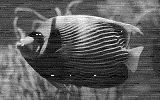64 point FFT, 21 carriers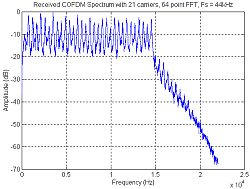(Click the image for a larger version)16 point FFT, 5 carriers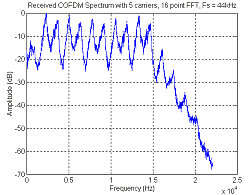(Click the image for a larger version)

Table 12 Received images from the audio tape player, using OFDM with 5 and 21 carriers.

#### 2.3.5.2 Tape Player Performance

The frequency stability and frequency response of the tape player were measured to assess the effective quality of the channel. All tests were performed using the audio tape player in conjunction with the Sound Blaster 16 card, thus the measurements are the combination of their performances

The measurements were performed using the same technique used for performance measurement of the VCR (see section 2.3.4.2).

#### Frequency Stability

A 10 kHz tone was generated using the sound card by playing a windows 3.1 WAV file generated by a Matlab script. This tone was then recorded on the tape player. The frequency and amplitude of the played back signal was then measured over a 10 second period. This time length was used because it was approximately the same length of time that is taken in transmitting the fish image. The results are shown below in Table 13

 Frequency (kHz) Amplitude Variation (dB) Max 10.000 0.21 Mean 9.987 0.01 Min 9.823 -0.25

Table 13 Frequency and amplitude fluctuations in a 10second, 10kHz tone played back from the audio tape player

Thus the frequency offset is -0.13% and the variation is 1.77%. The level of frequency instability is very high as even for only 21 carriers this represents the carrier frequencies shifting by up to 40% of a carrier spacing. This frequency instability would cause large phase errors to occur

#### Frequency Response

The tape player / Sound Blaster 16 frequency response is shown below in Figure 27. The bandwidth of the tape player is about 15 kHz, plus the response is reasonably flat (± 3 dB). The frequency response of the tape player should not cause any significant problems for the OFDM signalFigure 27 Record / Play back frequency response of the JVC TD-W444 / Sound Blaster 16 with a sample rate of 44.1kHz

## 2.4 Picture quality verse signal to noise ratio

This section describes some work that was done relating to improving the degradation performance of OFDM when the channel noise becomes very high. One problem with many digital communication systems is that the performance of the system is fine, up to some critical channel noise level, above which the system fails very quickly. This can cause potential problems for wireless telecommunications where the received signal quality can vary greatly depending of the location of the mobile station. This problem leads to drop outs in the signal, decreasing the reliability of the system. It would be far better if the system simply gave a worse voice or image quality under high noise conditions, rather then completely loosing the signal

It was noticed when doing the practical measurements on OFDM (see section 2.3) that the received quality of the image was very good using 256PSK even though the measured error rate was very high. Using 256PSK to transmit the image is similar to sending an analog transmission as channel noise simply appears as noise in the image. The main question was, does sending the image using 256PSK result in better performance then QPSK in a high noise environment? To answer this question, a simple comparison was set up

### 2.4.1 Setup

The same Matlab scripts were used for this test as in the previous practical measurements, however the signal wasn’t recorded to any external audio channel as in the practical tests. The channel noise was simulated using the same model as used in the OFDM simulations (see section 2.1). The signal to noise ratio (SNR) of the channel was varied from 0 dB up to 15 dB, with the image quality measured at 3 dB increments. No forward error correction was used for either modulation technique

Using 256PSK allows 8 bits to be sent on each carrier per symbol, compared to only 2 bits for QPSK. Thus, 256PSK on its own allows for up to 4 times the transmission data rate. Since the aim of the experiment was to compare the relative performance differences between QPSK and 256PSK under the same transmission bandwidth and data rate, the 256PSK transmission was slowed down by 4 times to have the same transmission rate. This was achieved by repeating the 256PSK symbols four times, thus reducing the data rate to the same as using QPSK. The four repetitions were then combined at the receiver by averaging the received demodulated phase angle. This averaging reduces the received phase noise by up to 6 dB.

Transmitting the signal using 256PSK with linear mapping of the pixel intensity is very similar to transmitting the imaging in an analog manner. This modulation technique is essentially linear Phase Modulation. Transmitting the signal in an analog manner result in a direct mapping between phase errors caused by the channel, and amplitude noise (pixel intensity) in the received image. Using a linear mapping tends to reduce the chance of the large catastrophic errors that can occur when sending digital data that is not mapped in a linear manner.

However when transmitting using a standard digital modulation technique such as QPSK, the SNR of the demodulated image can be much higher then the channel SNR, provided the channel SNR is greater then ~10 dB. This is because small phase errors are not big enough to cause a bit error and thus no noise is seen on the received signal. However when the SNR of the channel becomes worse (< 10 dB), phase errors cause the received signal to be misread thus causing bit errors. Since the mapping of the bits to the amplitude of the transmitted signal are not linearly related, a single bit error can cause a significant error in received amplitude. This results in a rapid drop in performance of the received signal as the SNR of the channel drops below ~10 dB for QPSK.

### 2.4.2 Results

Figure 28 shows how the signal to noise of the received image decreases as the signal to noise ratio of the transmission channel also worsens. It can be seen that the 256PSK signal performs better then the QPSK transmission below a channel SNR of 9 dB.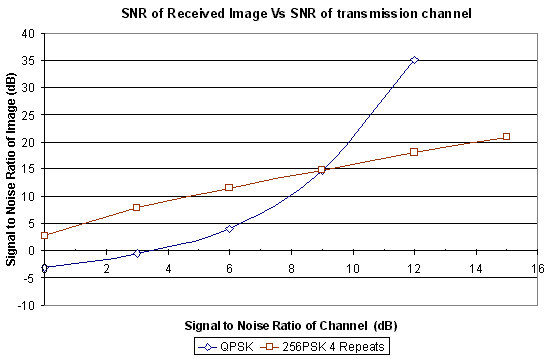Figure 28 Comparison between the received image SNR using QPSK and 256PSK verses the SNR.

For a channel SNR of 0 - 6 dB the 256PSK signal averaged approximately 7 - 9 dB better picture quality then the QPSK signal. This gain is to be expected as there are two reasons for the improvement. Firstly, the signal has been averaged (thus reducing the phase noise), and that the phase angle mapping used for 256PSK, as the 256PSK signal is averaged over 4 repeats, giving approximately a 6 dB improvement compared with the channel SNR.

Table 14 shows some of the received images. It is easy to see that the image quality of the signal transmitted using 256PSK is much better then using QPSK

 QPSK 256 PSK with 4 times data averagingSNR = 12dB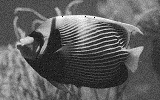SNR = 12dB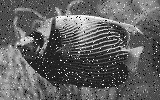SNR = 6dB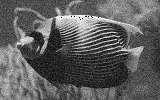SNR = 6dBSNR = 0dBSNR = 0dB

Table 14 Comparison between QPSK and 256PSK for transmitting an image under noisy conditions.

It was found that the picture sent using QPSK had a much better quality then the 256PSK signal in a low noise channel, where as the 256PSK performed better then QPSK under high noise conditions with a SNR < 9 dB.

Note: the performance of the QPSK signal can be significantly improved using advanced forward error correction techniques. This however cannot be applied to 256PSK using these same techniques as the matching between the phase mapping and the pixel intensity will be lost and the error is too high (typically 0.8-0.95 for SNR <12 dB) for most error correction to work successfully. The use of forward error correction techniques may result in the performance of QPSK and 256PSK being the same or QPSK performing better

## 2.5 Mathematical Model for OFDM performance

The aim was to develop a mathematical model of the performance (BER) of OFDM verses the channel noise. This was so that the simulated results could be verified, and to get a more in depth understanding of the transmission mechanism.

The model developed is based on the transmission modulation technique being phase shift keying, and that the channel noise is gaussian noise (i.e. white noise)

### 2.5.1 RMS Demodulated Phase Error

If we assume that the transmission modulation method used is phase shift keyed then any noise added to the transmitted signal will result in a phase error. If we look at the IQ diagram of the transmitted signal then the transmitted signal will be a phasor of fixed magnitude, and of phase corresponding to the data to be transmitted. The noise can then be considered as the random vector added to the transmitted signal. The magnitude of the phase error depends on two things, the relative phase angle of the noise vector, and the magnitude of the noise vector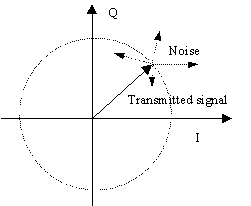The received vector will be the vector sum of the transmitted signal and the noise. If we assume that the noise is a constant magnitude vector equal to its RMS magnitude, and that it has a random phase angle then the problem of working out the received angle would be as follows

### 2.5.2 BER verses Channel Noise

Figure 29 show the effect of noise on the received phase angle. If we let the amplitude of the transmitted signal be 1, and the length of the noise vector be A with angle f, then the received phase error is θerr.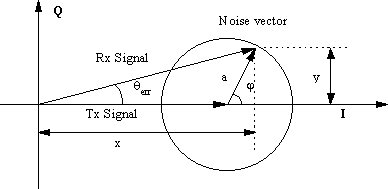Figure 29 Received Phasor, showing effect of noise on the received phase angle.

Using trigonometry,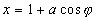Since,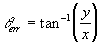Therefore,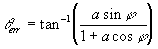The signal to ratio determines the relative amplitude of the received signal and the noise level. Since the signal is scaled to an amplitude of 1, the amplitude of the noise is: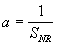Note: The SNR is base on the amplitudes of the signals thus must be scaled correctly when converting it to dB

If we substitute this in we get,The noise signal can be of any phase angle. What we need is to find is the RMS phase error, so if we find the average phase error (assuming the noise phase angle is always positive) then this can be scaled to find the RMS error. The average phase angle can be found by integrating θerrover a half circle, i.e j varies from 0 to p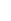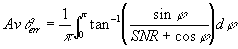The RMS phase error will be greater by root 2, thus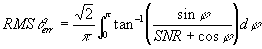Equation 1

This equation was was used to predict the RMS phase error for different channel SNRs. This was compared with the results obtained using the simulation of OFDM. Figure 30 shows that the predicted results based on the above mathematical derivation match the simulated results very wellFigure 30 Comparison between the measured RMS phase error using the simulations and the predicted result. (Also shown in Table 22)

Once the RMS phase error has been calculated the BER can be easily calculated using simple statistics. The RMS phase error is the standard deviation of the phase error. An error will occur if the phase error gets bigger then the maximum allowed for the modulation method used. Thus the BER can be determined by finding the probability of the phase error being greater the plus, minus the maximum phase error for a standard deviation equal to the RMS phase error. This is outlined in the following example

The Bit Error Rate (BER) of an OFDM link can be predicted based on the channel signal to noise ratio (SNR) and phase modulation used (e.g. BPSK, QPSK, etc). This is done by finding out what the expected RMS phase error (θerror(rms)) there will be on the signal (due to the channel noise). The bit error rate can then be found by comparing the magnitude of the RMS phase error to that of the maximum phase allowed for the particular phase modulation used (θmax).

### 2.5.3 Maximum Allowable Phase Angle

θmax is the maximum phase error allowed on the received symbol, before an error will occur on the received word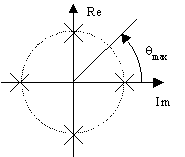Figure 31 IQ diagram for QPSK, showing the phase locations for data (crosses) and that θmax is 45 degrees

 Modulation Technique Maximum Phase Error Allowed θmax in degrees BPSK 90 QPSK 45 16PSK 11.25 256PSK 0.70313

Once θmax and θerror(rms) have been established, Z can be calculated, and the BER found from Table 22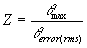#### Example

For a QPSK transmission if the signal to noise ratio (SNR) of the channel is 10dB, find the BER:

Note: Table 21 shows a summary calculated from Equation 1

From Table 21, θerror(rms)=16.5 degrees,

For QPSK,θmax=45 degrees,

Therefore,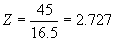From Table 22 the BER is between 0.0053 and 0.0091, with a result of 0.0077 if the results are interpolated

## 2.6 OFDM system implementation

The proposed final application for OFDM is to use it for wireless communications systems such as cellular mobile phone systems, fixed wireless phone systems, wireless data links and wireless computer local area networks. If OFDM is to be used in any of these applications then the bandwidth used must be sufficiently high to compete with other radio technologies.  This section discusses the processing power required to implement a practical OFDM system

An OFDM system mainly involves digital signal processing, thus the main focus of the performance of the system depends on the availability of high performance signal processing. There are two main ways in which the OFDM signal can be processed, which are using a general purpose DSP, or by implementing the processing in hardware using customized IC’s.

### 2.6.1 Using general purpose DSPs

There are several processing stages required to generate and receive an OFDM signal. However most of the processing is required in performing the Fast Fourier Transform (FFT).

The complexity of performing an FFT is dependent on the size of the FFT. The larger the FFT the greater the number of calculations required, however since as the symbol period is longer the increased processing required is less then the straight increase in processing to perform a single FFT. Table 15 shows the number of calculations required for an FFT (radix-2) of size N, and also the relative processing for various FFT sizes. It can be seen that because the symbol period increases with a larger FFT that the extra processing required is minimal

 Size FFT (N) Total number of complex calculations (values from ) Relative processing required for OFDM generation (normalized to 1024 point FFT) 32 240 0.5 64 576 0.6 128 1344 0.7 256 3072 0.8 512 6912 0.9 1024 15360 1.0 2048 33792 1.1 4096 73728 1.2

Table 15 Processing complexity for FFT

The processing efficiency of a DSP processor depends on the architecture of the processor, however for most single instruction DSP’s the number of cycles required to calculate an FFT is twice the total number of calculations shown in Table 15. This is due to complex calculations requiring two operations per calculation

#### Required Processing Power

To get an estimate of the processing power required to implement a practical phone system, lets consider an example

 Basic System Parameter Value Total Bandwidth 1.25MHz User Capacity 64 users Modulation Used QPSK FFT size 2048 Guard period 512 samples

Table 16 Example OFDM system

Table 16 shows a example system. From these basic system parameters the required number of carriers, user data rate and symbol rate can be calculated. These are shown in

 Derived System Parameter Value No. of active carriers 832 Data Rate of each User 39 kbps Useful symbol time 666 msec Total symbol time 833 msec

Table 17 Derived system parameters for the example OFDM system

From Table 15 the number of complex calculations required for a 2048 point FFT is 33792. The maximum time that can be taken in performing the calculation is once every symbol thus once every 833 msec. If we assume that the processor used requires 2 instructions to perform a single complex calculation, and that there is an overhead of 30% for scheduling of tasks and other processing. The minimum processing power required for this is then: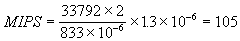Thus the transmitter requires >105MIPS in order to implement to OFDM transmitter. The receiver will require just as much as the transmitter, thus a full OFDM transceiver will require >210MIPS. This is a lot of processing required. Most current cheap DSPs are only 25-50MIPS (i.e. AD2181 is 33MIPS). Currently the fastest general purpose DSP is produced by Texas Instruments. The TMS320C62xx is capable of up to 1600MIPS which would make it plently fast enough for an OFDM transceiver. However, the price of the TSM320C62xx is not known and is expected to be very expensive

(Addendum 10/2001: Texas Instruments are now producing the TMS320C64xx, which is the next generation of fixed point DSP’s. These processors are capable of up to 4800 MIPS).

OFDM clearly requires a large amount of porcessing power, however since computer technology is advancing so fast this may not become a problem in the future

### 2.6.2 Future DSP Processing Power

Computer technology, and signal processing technology is improving at a rapid rate. The IC industry is on a self-prescribed course of development of ever-smaller devices and faster circuits. By the year 2000, logic IC will employ up to 60 million transistors and operate at speeds of over 1GHz.

Figure 32 shows the expected performance increase in general purpose microprocessors until the year 2010. If this is considered as an indicator for DSP improvement in speed, this shows that for the next 5 years we can expect a speed improvement in processing of greater than 5 times. Thus an OFDM system will be easily achievable using general purpose DSPs in 5 years time

(Addendum 10/2001: Texas Instruments announced during 1999 that they predict that by 2010, Digital Signal Processors will be capable of more than 1 Trillion Operations Per Second, www.ti.com/sc/docs/news/1999/99086.htm)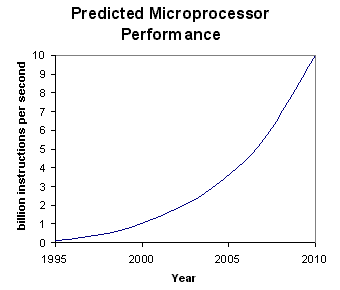Figure 32 The performance of general-purpose microprocessors will climb from 100 million operations per second in 1995 to more than 10 billion by 2010 (Source )

### 2.6.3 Hardware FFT Implementation

Another way of implementing the FFT processing required for generating and receiving the OFDM signal is by doing the FFTs in hardware using FFT IC’s. Several 1024 point FFT chips are shown in Table 18. For the previos example a 2048 point FFT needed to be performed in less then 830 msec. This is much greater then the execution time required by any of the FFT Ics in Table 18. Although these Ics only perform a 1024point FFT, clearly the processing can be easily achieved using hardware implementation of the FFT processing

 Processor Year Datapath Width (bits) Execution Time (1024-pt FFT) Number of Chips Required Pins Power (mW) Cobra (Colorada State) 1994 23 9.5 msec >16 391 7700 PDSP16510A (Plessey) 1989 16 98 msec 1 84 3000 DSP-24 (DSP Architectures) 1997 24 21 msec 7 308 3500+ Spiffe ULP (Stanford) 1995 20 61 msec 1 70 8

Table 18 1024 point FFT Chip Comparison

Next Chapter 3